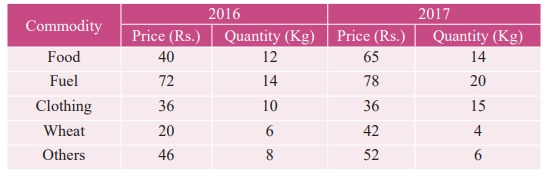Home | | Business Maths 12th Std | Exercise 9.2: Index Number

# Exercise 9.2: Index Number

Book back answers and solution for Exercise questions - Maths: Applied Statistics: Index Number: Problem Questions with Answer, Solution

Exercise 9.2

1. Define Index Number.

2. State the uses of Index Number.

3. Mention the classification of Index Number.

4. Define Laspeyre’s price index number.

5. Explain Paasche’s price index number.

6. Write note on Fisher’s price index number.

7. State the test of adequacy of index number.

8. Define Time Reversal Test.

9. Explain Factor Reversal Test.

10. Define true value ratio.

11. Discuss about Cost of Living Index Number.

12. Define Family Budget Method.

13. State the uses of Cost of Living Index Number.

14. Calculate by a suitable method, the index number of price from the following data:15. Calculate price index number for 2005 by (a) Laspeyre’s (b) Paasche’s method16. Compute (i) Laspeyre’s (ii) Paasche’s (iii) Fisher’s Index numbers for the 2010 from the following data.17. Using the following data, construct Fisher’s Ideal index and show how it satisfies Factor Reversal Test and Time Reversal Test?18. Using Fisher’s Ideal Formula, compute price index number for 1999 with 1996 as base year, given the following:19. Calculate Fisher’s index number to the following data. Also show that it satisfies Time Reversal Test.20. The following are the group index numbers and the group weights of an average working class family’s budget. Construct the cost of living index number:21. Construct the cost of living Index number for 2015 on the basis of 2012 from the following data using family budget method.22. Calculate the cost of living index by aggregate expenditure method:14. Laspeyre’s IN = 144.8 Paasche’s IN = 144.4

15. Laspeyre’s IN = 228.2 Paasche’s IN = 225.4

16. Laspeyre’s IN = 106.6 Paasche’s IN = 106.8 Fisher’s IN= 106.7

17. Fisher’s IN = 138.5 TRT= 1 FRT = 1880/1560

18. Fisher’s IN = 83

19. Fisher’s IN = 103 TRT = 1

20. Cost of Living Index =2662.38

21. Cost of Living Index = 117.31

22. Cost of Living Index = 131.49

Tags : Problem Questions with Answer, Solution | Applied Statistics , 12th Business Maths and Statistics : Chapter 9 : Applied Statistics
Study Material, Lecturing Notes, Assignment, Reference, Wiki description explanation, brief detail
12th Business Maths and Statistics : Chapter 9 : Applied Statistics : Exercise 9.2: Index Number | Problem Questions with Answer, Solution | Applied Statistics